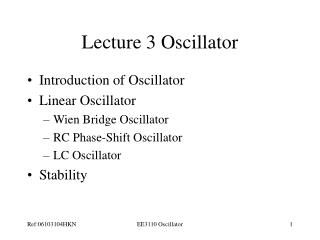Download PresentationLecture 3 Oscillator

# Lecture 3 Oscillator - PowerPoint PPT Presentation

Lecture 3 Oscillator. Introduction of Oscillator Linear Oscillator Wien Bridge Oscillator RC Phase-Shift Oscillator LC Oscillator Stability. Oscillators. Oscillation: an effect that repeatedly and regularly fluctuates about the mean value Oscillator: circuit that produces oscillationI am the owner, or an agent authorized to act on behalf of the owner, of the copyrighted work described.
Download Presentation## Lecture 3 Oscillator

Download Policy: Content on the Website is provided to you AS IS for your information and personal use and may not be sold / licensed / shared on other websites without getting consent from its author.While downloading, if for some reason you are not able to download a presentation, the publisher may have deleted the file from their server.

- - - - - - - - - - - - - - - - - - - - - - - - - - E N D - - - - - - - - - - - - - - - - - - - - - - - - - -
Presentation Transcript
1. Lecture 3 Oscillator • Introduction of Oscillator • Linear Oscillator • Wien Bridge Oscillator • RC Phase-Shift Oscillator • LC Oscillator • Stability EE3110 Oscillator

2. Oscillators Oscillation: an effect that repeatedly and regularly fluctuates about the mean value Oscillator: circuit that produces oscillation Characteristics: wave-shape, frequency, amplitude, distortion, stability EE3110 Oscillator

3. Application of Oscillators • Oscillators are used to generate signals, e.g. • Used as a local oscillator to transform the RF signals to IF signals in a receiver; • Used to generate RF carrier in a transmitter • Used to generate clocks in digital systems; • Used as sweep circuits in TV sets and CRO. EE3110 Oscillator

4. Linear Oscillators • Wien Bridge Oscillators • RC Phase-Shift Oscillators • LC Oscillators • Stability EE3110 Oscillator

5. Integrant of Linear Oscillators For sinusoidal input is connected “Linear” because the output is approximately sinusoidal A linear oscillator contains: - a frequency selection feedback network - an amplifier to maintain the loop gain at unity EE3110 Oscillator

6. Basic Linear Oscillator and If Vs = 0, the only way that Vo can be nonzero is that loop gain A=1 which implies that (Barkhausen Criterion) EE3110 Oscillator

7. Wien Bridge Oscillator Frequency Selection Network Let and Therefore, the feedback factor, EE3110 Oscillator

8.  can be rewritten as: For Barkhausen Criterion, imaginary part = 0, i.e., Supposing, R1=R2=R and XC1= XC2=XC, EE3110 Oscillator

9. Example By setting , we get Imaginary part = 0 and Due to Barkhausen Criterion, Loop gain Av=1 where Av : Gain of the amplifier Wien Bridge Oscillator Therefore, EE3110 Oscillator

10. RC Phase-Shift Oscillator • Using an inverting amplifier • The additional 180o phase shift is provided by an RC phase-shift network EE3110 Oscillator

11. Applying KVL to the phase-shift network, we have Solve for I3, we get Or EE3110 Oscillator

12. The output voltage, Hence the transfer function of the phase-shift network is given by, For 180o phase shift, the imaginary part = 0, i.e., Note: The –ve sign mean the phase inversion from the voltage and, EE3110 Oscillator

13. LC Oscillators • The frequency selection network (Z1, Z2 and Z3) provides a phase shift of 180o • The amplifier provides an addition shift of 180o Two well-known Oscillators: • Colpitts Oscillator • Harley Oscillator EE3110 Oscillator

14. For the equivalent circuit from the output Therefore, the amplifier gain is obtained, EE3110 Oscillator

15. The loop gain, If the impedance are all pure reactances, i.e., The loop gain becomes, The imaginary part = 0 only when X1+ X2+ X3=0 • It indicates that at least one reactance must be –ve (capacitor) • X1 and X2 must be of same type and X3 must be of opposite type With imaginary part = 0, For Unit Gain & 180o Phase-shift, EE3110 Oscillator

16. Hartley Oscillator Colpitts Oscillator EE3110 Oscillator

17. Colpitts Oscillator Equivalent circuit In the equivalent circuit, it is assumed that: • Linear small signal model of transistor is used • The transistor capacitances are neglected • Input resistance of the transistor is large enough EE3110 Oscillator

18. At node 1, where, Apply KCL at node 1, we have For Oscillator V must not be zero, therefore it enforces, EE3110 Oscillator

19. Imaginary part = 0, we have Real part = 0, yields EE3110 Oscillator

20. Frequency Stability • The frequency stability of an oscillator is defined as • Use high stability capacitors, e.g. silver mica, polystyrene, or teflon capacitors and low temperature coefficient inductors for high stable oscillators. EE3110 Oscillator

21. Amplitude Stability • In order to start the oscillation, the loop gain is usually slightly greater than unity. • LC oscillators in general do not require amplitude stabilization circuits because of the selectivity of the LC circuits. • In RC oscillators, some non-linear devices, e.g. NTC/PTC resistors, FET or zener diodes can be used to stabilized the amplitude EE3110 Oscillator

22. Wien-bridge oscillator with bulb stabilization EE3110 Oscillator

23. Wien-bridge oscillator with diode stabilization EE3110 Oscillator

24. Twin-T Oscillator EE3110 Oscillator

25. Bistable Circuit EE3110 Oscillator

26. A Square-wave Oscillator EE3110 Oscillator